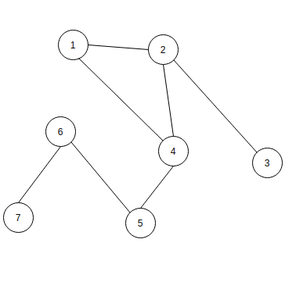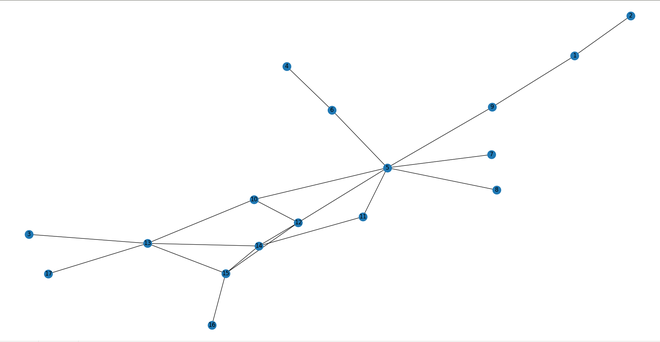# K-shell decomposition on Social Networks

• Last Updated : 01 Oct, 2020

Prerequisite: Introduction to Social Networks

K-shell decomposition is the method in which we can divide nodes on the basis of the number of its degree like nodes with degree 1 in one bucket etc.

Consider an example, assume there are n nodes and you apply k-shell decomposition in it. So nodes with degree 1 will be in bucket1 then we will see that after disconnecting these nodes is there any node left with degree 1 if yes then we will add them in bucket 1 and again check and repeat these steps for degree 2, 3, and so on and put them in bucket2, bucket3, etc.Initial Graph with 7 nodes

In the above graph first, we will put nodes with degree 1 in bucket 1 i.e node 3 and 7. After that, we will remove nodes 3 and 7 and check if there is any node left with degree 1 i.e node 6. Now we will remove node 6 and check any degree 1 node is left which is node 5. So we will remove node 5 and again check but there is no node left with degree 1, so now we will check for nodes with degree 2 which are nodes 1, 2, and 4 and now there is node left in the graph. So bucket1 = [3, 7, 6, 5] and bucket2 = [1, 2, 4].

Below is the implementation of K-shell decomposition on a Social Network:

## Python3

 `# Import required modules``import` `networkx as nx``import` `matplotlib.pyplot as plt`` ` ` ` `# Check if there is any node left with degree d``def` `check(h, d):``    ``f ``=` `0`  `# there is no node of deg <= d``    ``for` `i ``in` `h.nodes():``        ``if` `(h.degree(i) <``=` `d):``            ``f ``=` `1``            ``break``    ``return` `f`` ` ` ` `# Find list of nodes with particular degree``def` `find_nodes(h, it):``    ``set1 ``=` `[]``    ``for` `i ``in` `h.nodes():``        ``if` `(h.degree(i) <``=` `it):``            ``set1.append(i)``    ``return` `set1`` ` ` ` `# Create graph object and add nodes``g ``=` `nx.Graph()``g.add_edges_from(``    ``[(``1``, ``2``), (``1``, ``9``), (``3``, ``13``), (``4``, ``6``),``     ``(``5``, ``6``), (``5``, ``7``), (``5``, ``8``), (``5``, ``9``), ``     ``(``5``, ``10``), (``5``, ``11``), (``5``, ``12``), (``10``, ``12``), ``     ``(``10``, ``13``), (``11``, ``14``), (``12``, ``14``), ``     ``(``12``, ``15``), (``13``, ``14``), (``13``, ``15``), ``     ``(``13``, ``17``), (``14``, ``15``), (``15``, ``16``)])`` ` ` ` `# Copy the graph``h ``=` `g.copy()``it ``=` `1`` ` ` ` `# Bucket being filled currently``tmp ``=` `[]`` ` ` ` `# list of lists of buckets``buckets ``=` `[]``while` `(``1``):``    ``flag ``=` `check(h, it)``    ``if` `(flag ``=``=` `0``):``        ``it ``+``=` `1``        ``buckets.append(tmp)``        ``tmp ``=` `[]``    ``if` `(flag ``=``=` `1``):``        ``node_set ``=` `find_nodes(h, it)``        ``for` `each ``in` `node_set:``            ``h.remove_node(each)``            ``tmp.append(each)``    ``if` `(h.number_of_nodes() ``=``=` `0``):``        ``buckets.append(tmp)``        ``break``print``(buckets)`` ` ` ` `# Illustrate the Social Network ``# in the form of a graph``nx.draw(g, with_labels``=``1``)``plt.show()`

Output:

```[[2, 3, 4, 7, 8, 17, 16, 1, 6, 9], [11, 5, 10, 13, 12, 14, 15]]
```Graph with 17 nodes

My Personal Notes arrow_drop_up